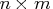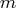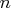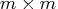Previous Page | Next Page

 Module Library

## CORR Function

computes correlation coefficients

CORR( matrix)

where matrix is anymatrix,is the number of variables, andis the number of data points.

The CORR function returns anmatrix of correlation coefficients. It computes the correlation between variables for any multivariate numeric data.Previous Page | Next Page | Top of Page